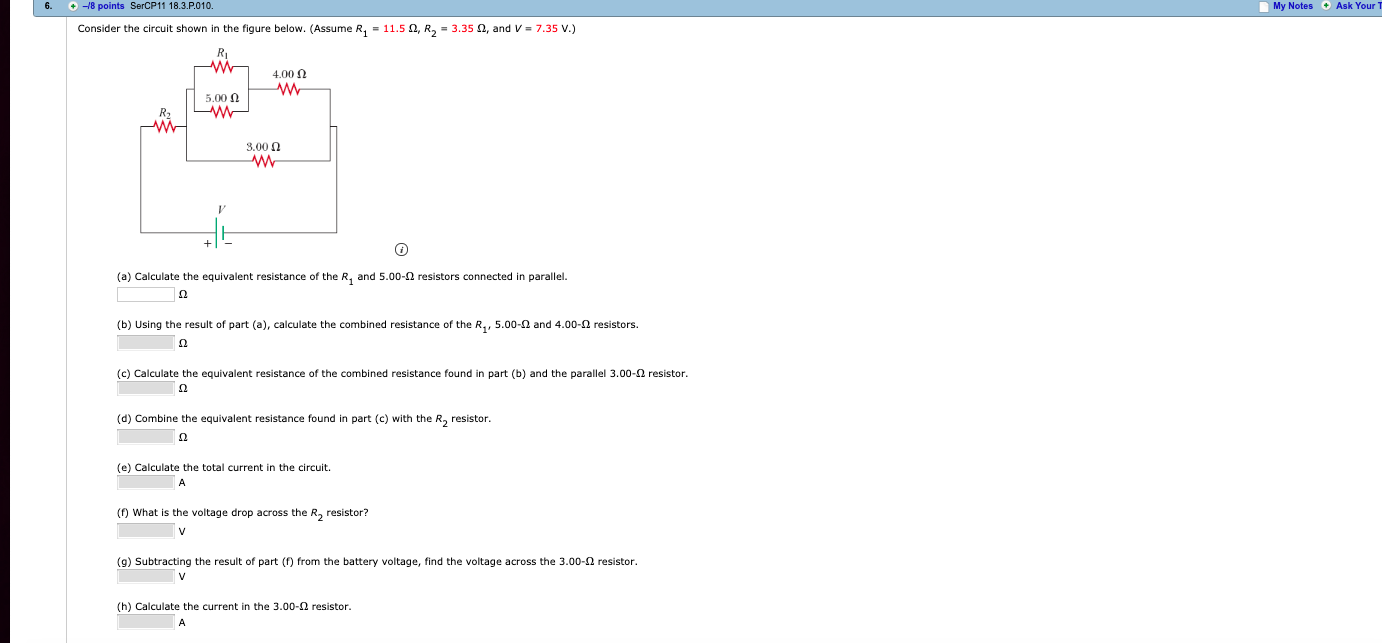# 6. 18 points SerCP11 18.3.P.010.My Notes Ask Your TConsider the circuit shown in the figure below. (Assume R1-11.5 Ω, R2-3.35 Ω, and V-7.35 V.)4,00 Ω5.00 Ω3.00 Ωw-(a) Calculate the equivalent resistance of the R1 and 5.00-Ω resistors connected in parallel.(b) Using the result of part (a), calculate the combined resistance of the R1, 5.00-Q and 4 00-Ω resistors.(c) Calculate the equivalent resistance of the combined resistance found in part (b) and the parallel 3.00-Ω resistor(d) Combine the equivalent resistance found in part (e) with the R2 resistor.(e) Calculate the total current in the circuit.(f) What is the voltage drop across the R2 resistor?(g) Subtracting the result of part (f) from the battery voltage, find the voltage across the 3.00-2 resistor(h) Calculate the current in the 3.00-Ω resistor

Question
88 viewshelp_outlineImage Transcriptionclose6. 18 points SerCP11 18.3.P.010. My Notes Ask Your T Consider the circuit shown in the figure below. (Assume R1-11.5 Ω, R2-3.35 Ω, and V-7.35 V.) 4,00 Ω 5.00 Ω 3.00 Ω w- (a) Calculate the equivalent resistance of the R1 and 5.00-Ω resistors connected in parallel. (b) Using the result of part (a), calculate the combined resistance of the R1, 5.00-Q and 4 00-Ω resistors. (c) Calculate the equivalent resistance of the combined resistance found in part (b) and the parallel 3.00-Ω resistor (d) Combine the equivalent resistance found in part (e) with the R2 resistor. (e) Calculate the total current in the circuit. (f) What is the voltage drop across the R2 resistor? (g) Subtracting the result of part (f) from the battery voltage, find the voltage across the 3.00-2 resistor (h) Calculate the current in the 3.00-Ω resistor fullscreen
check_circle

Step 1

(a)

The equivalent resistance of the resistor R1 and 5.00 Ω is,

Step 2

Substituting the values,

Step 3

(b)

The combined resistance of the resistors R...

### Want to see the full answer?

See Solution

#### Want to see this answer and more?

Solutions are written by subject experts who are available 24/7. Questions are typically answered within 1 hour.*

See Solution
*Response times may vary by subject and question.
Tagged in

### Current Electricity